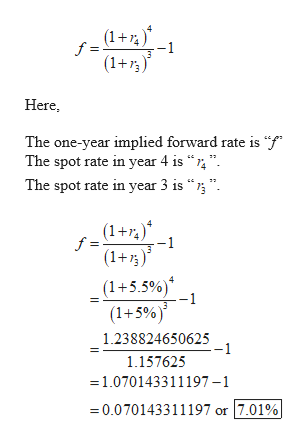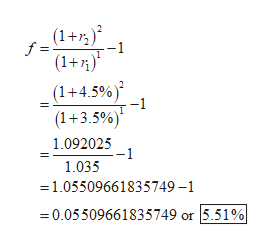# The Analysis and Valuation of Bonds, Investment Analysis and Portfolio Management1. The following table shows spot rates on US Treasury securities as of January 1, 2019:Term to Maturity CalculatedSpot Rates1 Year 3.50%2 Years 4.50%3 Years 5.00%4 Years 5.50%5 Years 6.00%10 Years 6.60%Based on the data on the tablea) Calculate the one-year implied forward rate, four years from now.b) Calculate the 2-year implied forward rate, two years from now.

Question

The Analysis and Valuation of Bonds, Investment Analysis and Portfolio Management
1. The following table shows spot rates on US Treasury securities as of January 1, 2019:
Term to Maturity Calculated
Spot Rates
1 Year 3.50%
2 Years 4.50%
3 Years 5.00%
4 Years 5.50%
5 Years 6.00%
10 Years 6.60%
Based on the data on the table
a) Calculate the one-year implied forward rate, four years from now.
b) Calculate the 2-year implied forward rate, two years from now.

check_circleExpert Solution
Step 1

a.

Calculate the one-year implied forward rate as follows:help_outlineImage Transcriptionclose(1+ f = (1 -1 Here, The one-year implied forward rate is "f" The spot rate in year 4 is "" The spot rate in year 3 is " (1+) (1+ f = -1 (1+5.5%) (1+5%) 1.238824650625 -1 1.157625 =1.070143311197-1 -0.070143311197 or 7.01% fullscreen
Step 2

b.

Calculate the two-year implied f...help_outlineImage Transcriptionclose(1+72) f = -1 (1+) (1+4.5%) -1 (1+3.5%) 1.092025 1.035 1.05509661835749-1 0.05509661835749 or 5.51% fullscreen

### Want to see the full answer?

See Solution

#### Want to see this answer and more?

Solutions are written by subject experts who are available 24/7. Questions are typically answered within 1 hour*

See Solution
*Response times may vary by subject and question
Tagged in